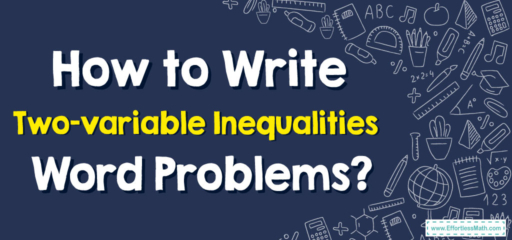# How to Write Two-variable Inequalities Word Problems?

Two-variable inequalities word problems involve using two variables and an inequality symbol to represent a relationship between two quantities in a real-world scenario. In these problems, there are two variables, often represented by $$x$$ and $$y$$, and an inequality symbol, such as $$"<"$$ (less than), $$">"$$ (greater than), $$"≤"$$ (less than or equal to), or $$"≥"$$ (greater than or equal to).## A Step-by-step Guide to Two-variable Inequalities Word Problems

Writing two-variable inequalities word problems involves several steps. Here’s a step-by-step guide:

### Step 1: Identify the variables

Identify the two variables that will be used in the inequality. It’s essential to define what each variable represents to avoid any confusion in the problem.

### Step 2: Determine the inequality symbol

Determine the inequality symbol that will be used in the problem. Common inequality symbols include “greater than,” “less than,” “greater than or equal to,” and “less than or equal to.” It’s important to select the correct symbol based on the problem’s context.

### Step 3: Write the inequality

Write the inequality using the variables and the inequality symbol.

### Step 4: Formulate the word problem

Write a word problem that uses the inequality. The word problem should be relevant to the inequality and include all necessary information, such as the values of the variables and any additional constraints.

### Step 5: Check for consistency

Check the word problem to ensure that it’s consistent with the inequality. The problem should be solvable using the inequality, and the solution should satisfy the inequality. If there are any inconsistencies, revise the problem or inequality as necessary.

### Writing Two-variable Inequalities Word Problems – Examples 1

John has $$500$$ to spend on shirts and pants. Shirts cost $$20$$ each, and pants cost $$30$$ each. Write an inequality that represents the possible combinations of shirts and pants John can buy.

#### Solution:

Step 1: Identify the variables

Let $$x$$ represent the number of shirts John can buy and $$y$$ represent the number of pants he can buy.

Step 2: Determine the inequality symbol

Since John has a fixed amount of money, the inequality will involve an inequality symbol that limits the total cost of the shirts and pants. The inequality symbol in this case is $$“≤”$$ (less than or equal to).

Step 3: Write the inequality

The inequality is: $$20x + 30y ≤ 500$$

Step 4: Formulate the word problem

How many shirts and pants can John buy with $$500$$ if he wants to spend all his money?

Step 5: Check for consistency

The word problem is consistent with the inequality.

### Writing Two-variable Inequalities Word Problems – Examples 2

Samantha is planning a trip to the beach and wants to rent beach chairs and umbrellas. She has a budget of $$200$$ and chairs cost $$10$$ each, while umbrellas cost $$20$$ each. Write an inequality that represents the possible combinations of chairs and umbrellas Samantha can rent.

#### Solution:

Step 1: Identify the variables

Let $$x$$ represent the number of chairs Samantha can rent and $$y$$ represent the number of umbrellas she can rent.

Step 2: Determine the inequality symbol

Since Samantha has a fixed amount of money, the inequality will involve an inequality symbol that limits the total cost of the chairs and umbrellas. The inequality symbol in this case is $$“≤”$$ (less than or equal to).

Step 3: Write the inequality

The inequality is: $$10x + 20y ≤ 200$$

Step 4: Formulate the word problem

How many chairs and umbrellas can Samantha rent with $$200$$ if she wants to spend all his money?

Step 5: Check for consistency

The word problem is consistent with the inequality.

### What people say about "How to Write Two-variable Inequalities Word Problems? - Effortless Math: We Help Students Learn to LOVE Mathematics"?

No one replied yet.

X
30% OFF

Limited time only!

Save Over 30%

SAVE $5 It was$16.99 now it is \$11.99